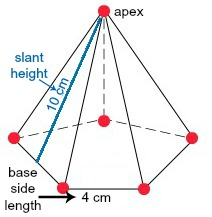# How to find the lateral area of a pentagonal pyramid

## Question:

How to find the lateral area of a pentagonal pyramid

## Lateral Area of a Pentagonal Pyramid:

In geometry, the lateral area of a three-dimensional object refers to the sum of the areas of the surfaces of the object excluding the areas of the bases. A pentagonal pyramid is a three-dimensional object with a pentagonal base and five triangular sides that meet at a point above the base, called the apex of the pyramid.

To find the lateral area of a pentagonal pyramid, we can find the area of each triangular side of the pyramid, and then add those areas up. However, if the pentagonal pyramid is regular, meaning that the sides of the pentagonal base all have the same length, then we have a nice formula we can use to find the lateral area of the pentagonal pyramid, which is as follows.

• Lateral Area = {eq}\frac{5}{2}(base \: side)(slant \: height) {/eq}

In this formula, base side refers to the length of one side of the base, and slant height refers to the distance from the apex of the pyramid to the midpoint of one of the sides of the base of the pyramid.

For instance, suppose we have a pentagonal pyramid that is regular as shown:We see that this pentagonal pyramid has a pentagonal base with sides of length 4 centimeters each, and the slant height of the pentagonal pyramid is 10 centimeters. We can find the lateral area of this pentagonal pyramid by plugging 4 in for the base side and 10 in for the slant height into our formula and evaluating.

• Lateral Area = {eq}\frac{5}{2}(base \: side)(slant \: height)=\frac{5}{2}(4)(10)=100 {/eq}

We get that the lateral area of our pentagonal pyramid is 100 square centimeters.How to Find Surface Area of a Pyramid

from

Chapter 2 / Lesson 7
15K

What is the surface area of a pyramid? Learn the definitions of pyramid and surface area, and see how to find the surface area of a pyramid with examples.